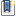ATOMS : Curve Fitters (curvefit) details

# Curve Fitters (curvefit)

To fit a given set of data points exponentially, linearly or to a polynomial.
Details
Version
1.0.1
Author
Manas Sharma
Owner Organization
Manas Sharma
Maintainer
Manas Sharma
Categories
Creation Date
March 14, 2016
Source created on
Scilab 5.4.x
Binaries available onThis toolbox is in the process of being built
Report a bugDescription
```            Toolbox: curvefit

Title: Curve Fitters

Summary: A toolbox for fitting data-points to a line, polynomial or an
exponential curve using the Least Square Approximation and plot the original and
fitted values.

Version: 1.0.1

Author: Manas Sharma

Maintainer: Manas Sharma

Category: Data Analysis and Statistics

Entity: Manas Sharma

WebSite: http://www.bragitoff.com/

ScilabVersion: >= 5.4

Depends:

Date: 2016-03-03

Description: A toolbox for fitting data-points to a line, polynomial or an
exponential curve using the Least Square Approximation.

Macros:

linefit - Fit a given set of data-points to a line. Returns the fitted data
points, slope and the intercept of the line.

npolyfit - To fit a given set of data points to a polynomial.

expofit - To Exponentially fit a given set of data points

lfitplot - Fit a given set of data-points to a line. Returns the fitted data
points, slope and the intercept of the line and plot the original and fitted
data..

pfitplot - To fit a given set of data points to a polynomial and plot the
original and fitted data..

efitplot - To Exponentially fit a given set of data points and plot the original
and fitted data.

HELP:
http://www.bragitoff.com/2016/03/exponential-fitting-scilab/
http://www.bragitoff.com/2016/03/linear-fitting-scilab/
http://www.bragitoff.com/2016/03/polynomial-fitting-scilab/            ```Files (1)Source code archive
```
```News (0)Comments (0)Leave a comment
Login withEmail notifications